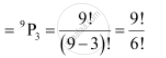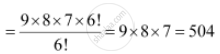CBSE (Arts) Class 11CBSE
Share

# How Many 4-digit Numbers Are There with No Digit Repeated? - CBSE (Arts) Class 11 - Mathematics

ConceptConcept of Permutations

#### Question

How many 4-digit numbers are there with no digit repeated?

#### Solution

The thousands place of the 4-digit number is to be filled with any of the digits from 1 to 9 as the digit 0 cannot be included. Therefore, the number of ways in which thousands place can be filled is 9.

The hundreds, tens, and units place can be filled by any of the digits from 0 to 9. However, the digits cannot be repeated in the 4-digit numbers and thousands place is already occupied with a digit. The hundreds, tens, and units place is to be filled by the remaining 9 digits.

Therefore, there will be as many such 3-digit numbers as there are permutations of 9 different digits taken 3 at a time.

Number of such 3-digit numbersThus, by multiplication principle, the required number of 4-digit numbers is 9 × 504 = 4536

Is there an error in this question or solution?

#### APPEARS IN

NCERT Solution for Mathematics Textbook for Class 11 (2018 to Current)
Chapter 7: Permutations and Combinations
Q: 2 | Page no. 148

#### Video TutorialsVIEW ALL 

Solution How Many 4-digit Numbers Are There with No Digit Repeated? Concept: Concept of Permutations.
S